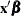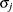# The QLIM Procedure

### OUTPUT Statement

• OUTPUT <OUT=SAS-data-set> <output-options> ;

The OUTPUT statement creates a new SAS data set containing all variables in the input data set and, optionally, the estimates of, predicted value, residual, marginal effects, probability, standard deviation of the error, expected value, conditional expected value, technical efficiency measures, and inverse Mills ratio. When the response values are missing for the observation, all output estimates except residual are still computed as long as none of the explanatory variables is missing. This enables you to compute these statistics for prediction. You can specify only one OUTPUT statement.

Details on the specifications in the OUTPUT statement are as follows:

CONDITIONAL

outputs estimates of conditional expected values of continuous endogenous variables.

ERRSTD

outputs estimates of, the standard deviation of the error term.

EXPECTED

outputs estimates of expected values of continuous endogenous variables.

MARGINAL

outputs marginal effects.

MILLS

outputs estimates of inverse Mills ratios of censored or truncated continuous, binary discrete, and selection endogenous variables.

OUT=SAS-data-set

names the output data set.

PREDICTED

outputs estimates of predicted endogenous variables.

PROB

outputs estimates of probability of discrete endogenous variables taking the current observed responses.

PROBALL

outputs estimates of probability of discrete endogenous variables for all possible responses.

RESIDUAL

outputs estimates of residuals of continuous endogenous variables.

XBETA

outputs estimates of.

TE1

outputs estimates of technical efficiency for each producer in the stochastic frontier model suggested by Battese and Coelli (1988).

TE2

outputs estimates of technical efficiency for each producer in the stochastic frontier model suggested by Jondrow et al. (1982).